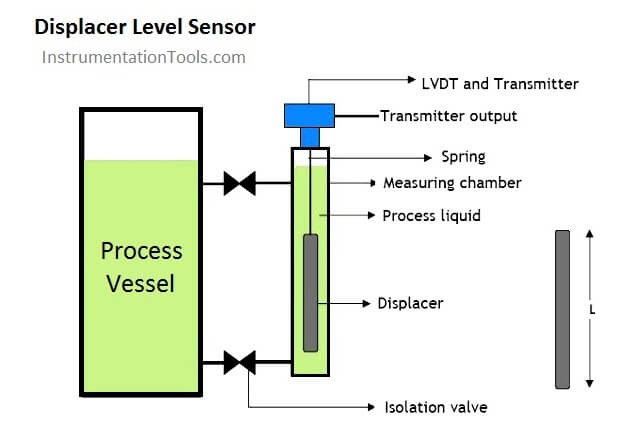# What is Displacer Level Sensor ?

Displacer level sensor use Archimedes’ Principle to detect liquid level by continuously measuring the weight of a displacer rod immersed in the process liquid.

The displacer is cylindrical in shape with a constant cross-sectional area and made long or short as required. Standard heights range from 14 inches to 120 inches.

As liquid level increases, the displacer rod experiences a greater buoyant force, making it appear lighter to the sensing instrument, which interprets the loss of weight as an increase in level and transmits a proportional output signal.

As liquid level decreases, the buoyant force on the displacer rod decreases with a corresponding weight increase which is interpreted as decreasing level by the level sensor which then give a corresponding signal output.

Shown below is a typical displacer level sensor installation:Although the basic theory of operation has been outlined above, in a practical displacer level sensor, the construction is engineered to achieve the desired measurement objective with sophisticated electronic circuitry.

In these types of displacer level sensors, the displacer is attached to a spring which restricts its movement for each increment of buoyancy (i.e. level change).

A transmitter incorporating a Linear Variable Differential Transformer (LVDT) is used to track the rise and fall of the displacer rod as liquid level changes. Sophisticated electronics is then used to process the voltage signal from the LDVT into a 4-20mA output signal.

Archimedes’ Principle Applied to the Displacer

According to Archimedes’ Principle, the buoyant force on an immersed object is always equal to the weight of the fluid volume displaced by the object.

Suppose in a displacer level sensor, we have a cylindrical displacer rod with density, ρ, radius, r, length, L, and process fluid of density, У. In this installation, the length of the displacer rod is proportional to the liquid level being measured:

Volume of displacer rod, V=πr2L

shown in the diagram for the displacer level sensor installation above, when the vessel is full, the displacer rod is completely immersed in the process fluid hence the volume of process fluid displaced is V=πr2L. When the level of the vessel is empty or minimum, volume of process fluid displaced is V = 0

Hence:

When vessel is full, buoyant force on displacer rod is given by:

Buoyant Force = weight of process fluid displaced

= πr2LУg (g = acceleration due to gravity)

Real weight of displacer = πr2Lρg

Net weight of displacer sensed by the LVDT, transmitter and associated electronics when vessel is full is:

= πr2Lρg−πr2LУg=πr2Lg(ρ–У)=Vg(ρ–У)

As can be seen above, the net weight sensed by the LVDT is proportional to the difference in density of the displacer rod (ρ) and that of the process fluid (У)

Therefore, the displacer rod must have a higher specific gravity than that of the liquid level being measured and have to be calibrated for the specific gravity of the liquid.

Typical specific gravity range for liquids where the displacer level sensor is applied is in the range of 0.25 to 1.5. Another point worth mentioning is that the range of the displacer level instrument is dependent only upon the length (L) of the displacer rod specified for the given application.

When vessel is empty or level is minimum,

Buoyant force on displacer = 0

Hence, weight sensed by the LVDT is =πr2Lρg

The LVDT registers a voltage signal at minimum vessel level and outputs a corresponding signal. The displacer length is determined by the operating range (span) specified, the specific gravity, pressure, and temperature of the process fluid. The diameter and weight are factory calculated to ensure correct operation and providing accurate 4-20mA output.

Areas of Application

The displacer level sensor is used in level measurement applications such as knock- out pots, condensate drums, separators, flash vessels, storage vessels and receiver tanks.